# Switching Angles Calculation in Multilevel Inverters Using Triangular Number Sequence –A THD Minimization Approach

Switching Angles Calculation in Multilevel Inverters Using Triangular Number Sequence –A THD Minimization Approach

Jesus Aguayo-Alquicira Susana Estefany De León-AldacoJorge Hugo Calleja-Gjumlich Abraham Claudio-Sánchez

Electronics Department, TecNM / Cenidet, Cuernavaca Morelos, 62490, México

Industrial Engineering Department, UNINI, Campeche Campeche, 24560, México

Corresponding Author Email:
Susana.da@cenidet.tecnm.mx
Page:
49-55
|
DOI:
https://doi.org/10.18280/ejee.220106
12 August 2019
|
Accepted:
20 November 2019
|
Published:
15 March 2020
| Citation

OPEN ACCESS

Abstract:

Many problems in nature can be solved by resorting to numbers in a particular sequence. For example, the numbers in the so called Fibonacci sequence have been successfully applied in computer science, mathematics and game theory. Another example is the triangular number sequence, which has two main applications: the handshake problem and the round-robin tournament. These two sequences can be found in the Pascal's triangle. With the aim of enhancing the waveform quality at the output of a multilevel inverter, this paper explores the suitability of the triangular number sequence to compute the commutation angles in Pulse Width Modulation switching pattern. It has been found that this approach provides a harmonic performance comparable to the results obtained with other calculation techniques such as the Newton Raphson method or Genetic Algorithm, but without the difficulty of solving the complex non-linear equation.

Keywords:

total harmonic distortion, pulse modulation, pascal triangle, triangular numbers, switching pattern

1. Introduction

Nowadays, Cascaded H-Bridge Multilevel Inverters (CMLI) are widely used in industrial applications mainly due to two advantages. The first one is that the difficulty involved in developing high-voltage, high-power inverters is greatly simplified, because the electrical stresses are distributed among several switching devices [1, 2]. The second one is that the stepped output simplifies the task of generating high-quality waveforms [3, 4], whose harmonic content is often expressed in terms of the total harmonic distortion (THD), as follows:

$T H D=\frac{\sqrt{\sum_{j=2}^{n} V_{j}}}{V_{1}}$        (1)

where, Vj corresponds to the amplitude of the j’th harmonic, and V1 is the fundamental amplitude.

Figure 1 shows the configuration of a seven-level CMLI, using three individual H bridges.where, Vj corresponds to the amplitude of the j’th harmonic, and V1 is the fundamental amplitude.

The modulation methods used to synthesize the output waveforms in multilevel inverters can be grossly classified as follows:

(1) Carrier-based techniques, extensions of the triangular waveform carrier technique used in low power inverters. Two alternatives are commonly used: in the carrier disposition approach (used in neutral-point-clamped multilevel inverters ) the triangular waveforms are arranged one on top of the other. The second alternative is applied in cascaded multilevel inverters and involves phase-shifted carrier waveforms. In both alternatives, the power stages are switched at the carrier frequency, thus contributing to power losses .

(2) Space vector PWM, a mathematical approach that also involves high-frequency switching in the power stages .

(3) Stepped waveform modulation, which offers the advantage of low-frequency switching, thus minimizing the losses in the power stages. In the selective harmonic elimination approach (SHEPWM) , the switching angles are calculated by solving a set of non-linear equations, in such a manner that several specific harmonics are eliminated .

## 1.jpg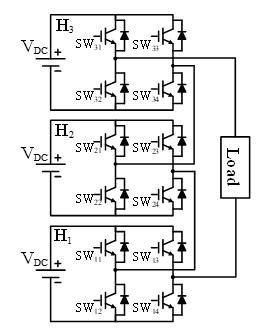Figure 1. Cascaded H bridge multilevel inverter (7-levels)

In the stepped waveform modulation, the number of harmonics to eliminate depends on the number of switching angles per quarter cycle . Thus, the complexity of the mathematical procedure increases as the quality of the output waveform is improved.

An alternative is to calculate the switching angles in such a manner that the overall THD is minimized . In comparison with other modulation methods, this approach provides an acceptable performance with low switching frequency to fundamental frequency ratios. Its main disadvantage, again, is that the suitable switching pattern calculation is a fairly complicated mathematical procedure.

Several mathematical approaches for switching pattern calculation have already been proposed in the technical literature. The Newton-Raphson (NR) based methods, for instance, are fast but do not yield correct solutions for all the possible modulation index values . Further, they depend on an initial guess and do not yield an optimal solution. The Genetic-Algorithm (GA) based methods are not as fast, but they are capable of providing the solutions to minimize the THD . All the approaches described above require the solution of a set of non-linear equations and the knowledge of complex computational algorithms to obtain the switching pattern.

In this paper, the switching angles for a multilevel inverter’s output are computed employing the triangular number sequence (TNS) extracted from Pascal’s triangle, which provides a simpler and faster computation method. It is assumed that the waveform has quarter-wave symmetry, an odd number of levels, and equal-height steps and one switching angles per level. The final goal is to enhance the output waveform quality, in such a way that a minimum THD is obtained.

The solution procedure does not require solving the complex non-linear equation obtained from the analysis of the output voltage waveform. This approach focuses on a multilevel inverter waveform with quarter-wave symmetry having equal-height steps, which implies equal DC voltage sources values. It uses a diagonal Pascal’s triangle sequence (triangular numbers) to determine the value of the switching pattern in a multilevel inverter. This method involves a simple sequence and can be used with multilevel inverters having an odd number of levels.

The method can be used to obtain the switching angles of a multilevel inverter of "n" levels by using triangular numbers in Uninterruptible Power Supply (UPS) applications that require a fixed frequency and a fixed modulation index and to obtain the best use of the CD bus with a low THD.

This paper is organized as follows. The problem statement is reviewed in Section 2. The triangular number sequence is presented in Section 3. Comparative results using several methods are illustrated in Section 4. Experimental results using triangular number sequence are presented in Section 5. Some conclusions are given in Section 6.

2. Problem Statement

Figure 2 shows the output voltage waveform V0(ωt) from a 7-level inverter. The waveform has both odd and quarter-wave symmetry, and its Fourier series is given by:

$V_{0}(\omega t)=\sum_{n=1}^{\infty}\left(b_{n} \sin (n \omega t)\right)$    (2)

With n odd. Fourier coefficients bn can be expressed as:

$b_{n}=\frac{4 V_{D C}}{n \pi}\left(\cos \left(n \alpha_{1}\right)+\left(\cos \left(n \alpha_{2}\right)+\left(\cos \left(n \alpha_{3}\right)\right)\right.\right.$    (3)

where, VDC is the height of each step, and α1, α2, and α3 are the switching angles.

## 3.jpg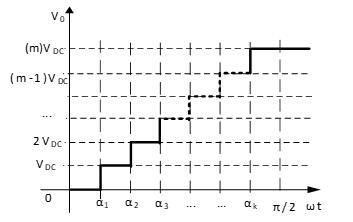Figure 2. Output voltage waveform in the multilevel inverter (7-levels)

The Fourier series can be expressed as:

${{V}_{0}}(\omega t)=\sum\limits_{n}^{\infty }{\left( \frac{4{{V}_{DC}}}{n\pi }(\cos (n{{\alpha }_{1}})+(\cos (n{{\alpha }_{2}})+(\cos (n{{\alpha }_{3}})) \right)\sin (n\omega t)}$    (4)

The THD value is determined with the values obtained from Eq. (3), and substituting in Eq. (1):

$THD=\frac{\sqrt{\sum\limits_{n=3(only\ odd)}^{\infty }{{{\left( \frac{4{{V}_{DC}}}{n\pi }(\cos (n{{\alpha }_{1}})+(\cos (n{{\alpha }_{2}})+(\cos (n{{\alpha }_{3}})) \right)}^{2}}}}}{\frac{4{{V}_{DC}}}{\pi }(\cos ({{\alpha }_{1}})+(\cos ({{\alpha }_{2}})+(\cos ({{\alpha }_{3}}))}$    (5)

By simplifying Eq. (5):

$THD=\frac{\sqrt{\sum\limits_{n=3(only\ odd)}^{\infty }{{{\left( \frac{1}{n}(\cos (n{{\alpha }_{1}})+(\cos (n{{\alpha }_{2}})+(\cos (n{{\alpha }_{3}})) \right)}^{2}}}}}{(\cos ({{\alpha }_{1}})+(\cos ({{\alpha }_{2}})+(\cos ({{\alpha }_{3}}))}$    (6)

The THD depends directly on the switching pattern in a multilevel inverter output waveform, and to reduce its magnitude it is necessary to obtain a set of switching angles, such that Eq. (6) is minimized .

The above procedure can be generalized for a waveform with m levels, equal height steps, as shown in Figure 3. The coefficients bn can be expressed as.

${{b}_{n}}=\frac{4{{V}_{DC}}}{n\pi }\sum\limits_{i=1}^{m}{\left( \cos (n{{\alpha }_{i}}) \right)}$    (7)

## 3.jpgFigure 3. m-level multilevel inverter output waveform with odd quarter-wave symmetry

From Eq. (2), The Fourier series can be expressed as:

${{V}_{0}}(\omega t)=\sum\limits_{n=1}^{\infty }{\left( \frac{4{{V}_{DC}}}{n\pi }\sum\limits_{i=1}^{m}{{{k}_{i}}\cos (n{{\alpha }_{i}})}) \right)\sin (n\omega t)}$    (8)

The THD value is obtained with:

$THD=\frac{\sqrt{\sum\limits_{n=3(only\ odd)}^{\infty }{\left( \frac{1}{n}\sum\limits_{i=1}^{m}{\cos (n{{\alpha }_{i}})} \right)}}}{\sum\limits_{i=1}^{m}{\cos ({{\alpha }_{i}})}}$    (9)

where, VDC is the voltage source, m is the numbers of levels, and αi are the switching angles.

It is possible to determine the switching pattern without solving the complex non-linear equation by applying a simple sequence of triangular numbers, these numbers have the characteristic of providing the switching pattern in multilevel inverters and achieving a reduced THD value.

3. Triangular Number Sequence (TNS)

3.1 What is a Triangular Number (TN)?

Triangular number or triangle number counts objects arranged in an equilateral triangle [14, 15]. The nth triangular number is the number of dots in the triangular arrangement with n dots on a side and is equal to the sum of the n natural numbers from 1 to “n”. The triangular number is a positive integer that can be represented by an equilateral triangular array.

The triangular numbers can be determined using the following expression:

$T{{N}_{n}}=\sum\limits_{k=1}^{n}{k}=\frac{n(n+1)}{2}$     (10)

where, TN is the triangular number and k is a constant value from 1 to “n”.

3.2 Example of TNS application for the calculation of a switching pattern for a seven-level CMLI

The switching angles (angles α1, α2, …, αk), can be obtained by way of the TNS where “k” is the number of angles to calculate.

Step 1: Determine the triangular number “n=k+1”.

$T{{N}_{k+1}}=\frac{(k+1)\left[ (k+1)+1 \right]}{2}=\frac{{{k}^{2}}+3k+2}{2}$     (11)

Step 2: Since an output signal with quarter-wave symmetry is required and a quarter-wave is equal to π/2. The value of π/2 is divided by the value obtained in the previous step, as follow:

${{F}_{\alpha }}=\frac{{}^{\pi }/{}_{2}}{T{{N}_{k+1}}}=\frac{{}^{\pi }/{}_{2}}{{}^{({{k}^{2}}+3k+2)}/{}_{2}}=\frac{\pi }{{{k}^{2}}+3k+2}$    (12)

Step 3: Each triangular number, from 1 to k, must be multiplied by the value obtained in step 2. These results correspond to the desired angles.

${{\alpha }_{1}}=T{{N}_{1}}{{F}_{\alpha }}$     (13)

${{\alpha }_{2}}=T{{N}_{2}}{{F}_{\alpha }}$      (14)

${{\alpha }_{k}}=T{{N}_{k}}{{F}_{\alpha }}$       (15)

For example, in a 7-level multilevel inverter, there is three switching angles “k=3” that can be calculated as follow:

a) Determine the triangular number “n=k+1=3+1”.

$T{{N}_{3+1}}=\frac{{{3}^{2}}+3\cdot 3+2}{2}=\frac{20}{2}=10$      (16)

b) Divide π/2 value between the value obtained in a).

${{F}_{\alpha }}=\frac{{}^{\pi }/{}_{2}}{10}=\frac{\pi }{20}=9{}^\circ$       (17)

c) Each triangular number, from 1 to k, must be multiplied by the value obtained in b). These results correspond to the desired angles.

${{\alpha }_{1}}=T{{N}_{1}}{{F}_{\alpha }}=(1)\left( \frac{\pi }{20} \right)=\frac{\pi }{20}=9{}^\circ$        (18)

${{\alpha }_{2}}=T{{N}_{2}}{{F}_{\alpha }}=(3)\left( \frac{\pi }{20} \right)=\frac{3\pi }{20}=27{}^\circ$       (19)

${{\alpha }_{3}}=T{{N}_{3}}{{F}_{\alpha }}=(6)\left( \frac{\pi }{20} \right)=\frac{6\pi }{20}=54{}^\circ$         (20)

3.3 Using the TNS approach as a function of the number of levels in the CMLI

The proposed TNS approach can be used for n-levels Cascaded Multilevel Inverter, without being limited to the 7-level as presented in the previous example (section III-B). The approach is valid from three-levels CMLI, and it is possible to use it until the physics of the implementation allows it or limits it.

## 4.jpg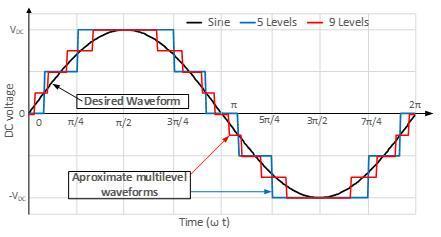Figure 4. Sine waveform as well as the 5-levels and 9-levels waveforms obtained with triangular numbers

Figure 4 shows the sine waveform, the 5-level and 9-level waveforms obtained with the TNS approach. The 5-level waveform is slightly approximated to sine waveform reducing THD. The 9-level waveform approximation to a sine waveform is higher than the 5-level waveform. In a multilevel inverter, when the number of levels increases, the output voltage THD decreases. The disadvantage of increasing the number of levels is that it increments the number of devices, hardware, and the control circuit becomes very complicated.

4. Comparative Results Using: Newton-Raphson, Genetic Algorithm and Triangular Numbers

In order to know the potential of application of the proposed approach based on TNS, a comparison of the results obtained with the classical Newton-Raphson method (NR) and a metaheuristic method such as Genetic Algorithm (GA) was carried out. The objective was to solve equation (9) to minimize THD value. The equation was adapted according to the number level of the CMLI, which vary from three to nine-levels.

Table 1 shows the comparative results obtained, between the NR and GA methods versus the proposed approach based on TNS. In addition, the table includes the value of "m" which represents the amplitude of the fundamental frequency component, the modulation index cannot be controlled with the proposed approach. Looking at Table 1, the results show that the values of the angles obtained using the three methods are very similar, with slight variations. However, the value of "m" is slightly lower using the proposed approach based on TNS.

Table 1. Switching angles comparison for different levels using TNS, NR & GA

 Levels TNS NR GA 3 α1=30° (m=1.10) α1=23.75° (m=1.17) α1=23.79° (m=1.17) 5 α1=15°, α2=45° (m=1.07) α1=13.38°, α2=43.05° (m=1.08) α1=13.41°, α2=41.91° (m=1.09) 7 α1=9°,  α2=27°, α3=54° (m=1.05) α1=9.22°, α2=27.97°, α3=52.01° (m=1.06) α1=9.12°, α2=27.94°, α3=51.43° (m=1.06) 9 α1=6°, α2=18°, α3=36°, α4=60° (m=1.04) α1=6.77°, α2=20.01°, α3=36.76°, α4=58.71° (m=1.04) α1=6.85°, α2=20.75°, α3=35.96°, α4=58.01° (m=1.04)

Table 2 presents the comparison of the THD value using the TNS, GA and NR methods. The results obtained in Table 2 shows that the values with any method are very similar with a slightly higher value when using TNS.

Table 2. THD value (%) comparison for different levels using TNS, NR & GA

 Levels TNS NR GA 3 30.0 27.91 27.90 5 15.84 15.37 15.30 7 10.72 10.49 10.47 9 8.67 8.00 7.86

Table 3 presents the comparison of the estimated number of floating point operations per second (FLOPS) value using the TNS, GA and NR methods. Using a computer with a CPU frequency: 2.536 GHz, number of CPUs: 1, number of cores: 4, number of threads: 4, having approximately 5 GFLOPS (109 FLOPS). The value of the time ("t") is presented in brackets in the same table, which represents the time in second to obtaining a result using the several methods, shows that the value of t value is nearly zero for the TNS method.

Figure 5 shows the harmonic values from fundamental to 50th harmonic using the TNS, GA and NR methods for a 7-level CMLI. The zoom in Figure 5 shows that the proposed focus allows the elimination of several harmonics (5th, 15th, 25th, 35th, and 45th), the NR and GA methods do not have this feature and show values for all harmonics. However, some harmonic values in TNS method are higher (13th, 23th, and 39th) than the values of the harmonics obtained by the NR and GA methods.

Table 3. Flops value comparison for different levels using TNS, NR & GA

 Levels TNS NR GA 3 1 (t=0.2ns) 11.67x109 (t=2.3s) 295.30x109 (t=59.0s) 5 3 (t=0.6ns) 12.47x109 (t=2.5s) 594.97x109 (t=119s) 7 5 (t=1ns) 28.85x109 (t=5.7s) 723.71x109 (t=144s) 9 7 (t=1.4ns) 71.44x109 (t=14.3s) 1,172.26x109 (t=234s)

## 5.jpg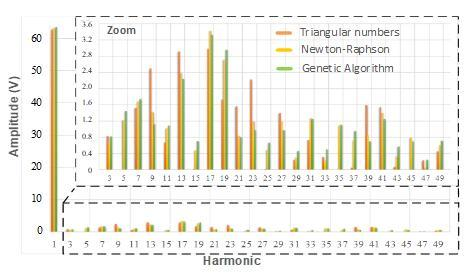Figure 5. Harmonics comparison of several methods for a 7-level CMLI

5. Experimental Results Using TNS

A modular cascade multilevel inverter was built in order to obtain the behavior of the proposed approach using TNS, obtaining a stepped output voltage from 3 to 9-levels. A configuration of individual H-bridges was used, from one bridge for three-levels to four H-bridges for nine-levels. Figure 6 shows a cell of the CMLI, using an IRAMS10UP60A module with their respective driver circuits, was implemented with 20V DC voltage supply. The switching angles obtained were implemented the FPGA of the ALTERA brand, using look-up tables for an off-line application.

Figure 7 shows the waveform of the output voltage obtained experimentally using the proposed approach, one H bridge 3-levels are obtained with one switching angles for each quarter-wave cycle, obtained in section IV (Table 1 in a second row).

Figure 8 shows the table of harmonic values from fundamental to 50th harmonic. A comparison between the THD values, the experimentally obtained (THD=29.81%) and the THD value showed in Table 2 (in the second row THD=30%), shows that both have the same value.

## 6.jpg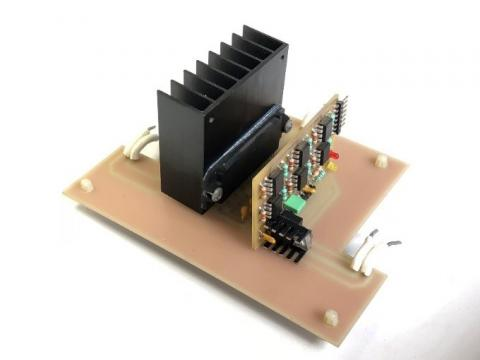Figure 6. Experimental H bridge circuit using IRAMS10UP60A module with its driver circuit

## 7.png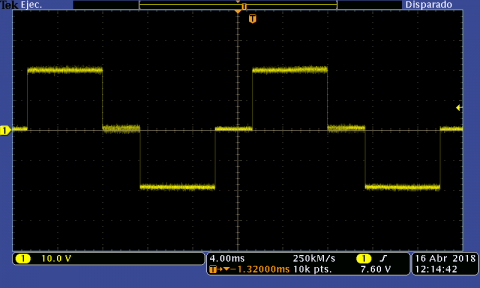Figure 7. Experimental result in a 3-level inverter using TNS for the switching angles

## 8.png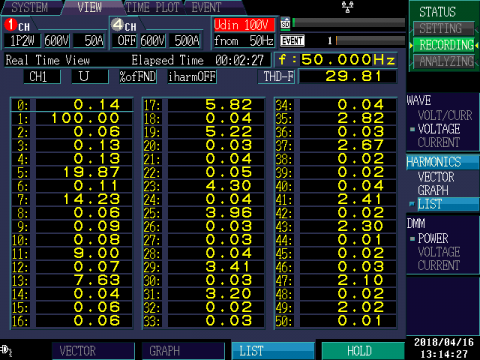Figure 8. Harmonic list for the experimental result in a 3-level using TNS

Figure 9 shows the waveform of the output voltage obtained experimentally using the proposed approach, two H bridge for 5-levels are obtained with two switching angles for each quarter-wave cycle, obtained in section IV (Table 1 third row).

Figure 10 shows the table of harmonic values from fundamental to 50th harmonic. A comparison between the THD values, the experimentally obtained (THD=15.71%) and the THD value showed in table 3 (in the third row THD=15.84%), shows that both have the same value.

Figure 11 shows the waveform of the output voltage obtained experimentally using the proposed approach, three H bridge for 7-levels are obtained with three switching angles for each quarter-wave cycle, obtained in section IV (Table 1 fourth row).

Figure 12 shows the table of harmonic values from fundamental to 50th harmonic. A comparison between the THD values, the experimentally obtained (THD=10.61%) and the THD value showed in Table 2 (in the fourth row THD=10.72%), shows that both have the same value.

## 9.png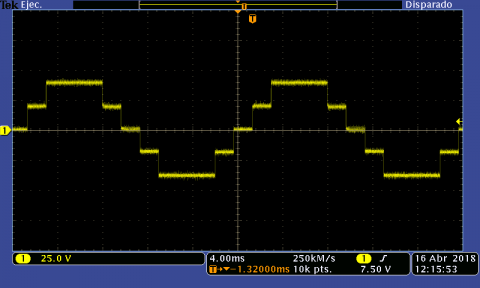Figure 9. Experimental result in a 5-level inverter using TNS for the switching angles

## 10.png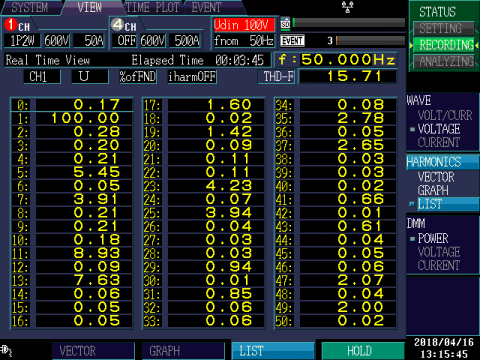Figure 10. Harmonic list for the experimental result in a 5-level using TNS

## 11.png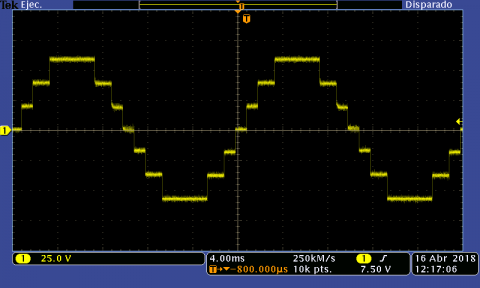Figure 11. Experimental result in a 7-level inverter using TNS for the switching angles

Figure 13 shows the waveform of the output voltage obtained experimentally using the proposed approach four H bridge for 9-levels are obtained with four switching angles for each quarter-wave cycle, obtained in section 4 (Table 1 at the bottom).

Figure 14 shows the table of harmonic values from fundamental to 50th harmonic. A comparison between the THD values, the experimentally obtained (THD=8.52%) and the THD value showed in Table 2 (at the bottom THD=8.67%), shows that both have the same value.

## 12.png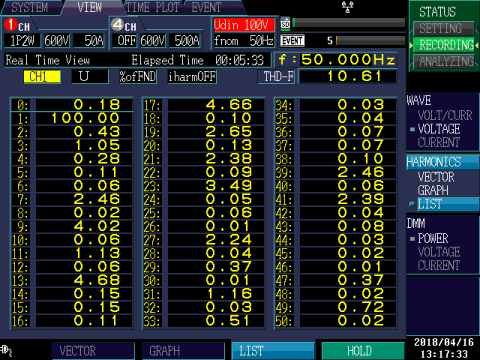Figure 12. Harmonic list for the experimental result in a 7-level using TNS

## 13.png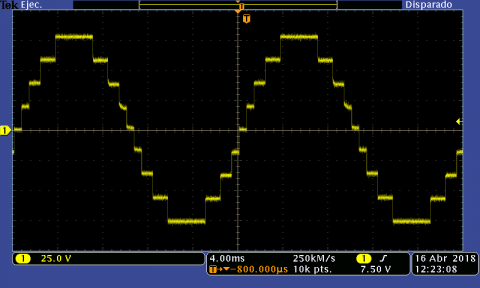Figure 13. Experimental result in a 9-level inverter using TNS for the switching angles

## 14.png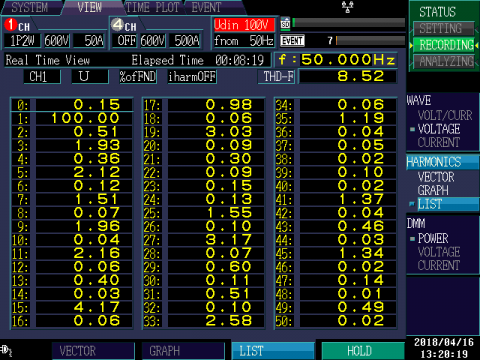Figure 14. Harmonic list for the experimental result in a 9-level using TNS

6. Conclusions

The triangular number sequence positioned in Pascal's triangle can be used to determine the suitable switching pattern in a multilevel inverter with the aim to reduce the Total Harmonic Distortion. This mathematical technique that uses the triangular number sequence to obtain the switching pattern is a quick and effective approach. Furthermore, it does not solve the non-linear equations that are obtained directly from the formal mathematical equations.

The goal is to avoid the time-consuming process of solving a non-linear equation. The results achieved demonstrate that the triangular number sequence approach to calculating the switching pattern in a multilevel inverter is a simple, easy to implement an alternative to the Newton-Raphson or Genetic Algorithm methods, which must solve the non-linear equation. The results also indicate that the triangular numbers approach provides a fast method of obtaining de switching angles, with almost the same value of THD.

This approach was used to resolve the switching patterns of the inverters from three to nine level, but the approach can be applied to any number of levels. The results showed that the output voltage THD is slightly larger in comparison with THD values obtained by the Genetic Algorithm or Newton-Raphson method.

References

 Franquelo, L.G., Rodríguez, J., Leon, J.L., Kouro, S., Portillo, R., Prats, M.A.M. (2008). The age of multilevel converters arrives. IEEE Industrial Electronics Magazine, 2(2): 28-39. https://dio.org/10.1109/MIE.2008.923519

 Alamri, B., Darwish, M. (2015). Power loss investigation in HVDC for cascaded H-bridge multilevel inverters (CHB-MLI). IEEE Eindhoven PowerTech, Eindhoven, pp. 1-7. https://doi.org/10.1109/PTC.2015.7232810

 Tarafdar Hagh, M., Najaty Mazgar, F., Roozbehani, S., Jalilian, A. (2017). THD minimisation of multilevel inverter with optimised dc sources magnitude and switching angles. CIRED - Open Access Proceedings Journal, 2017(1): 875-878. https://doi.org/10.1049/oap-cired.2017.0341

 Halim, W.A., Rahim, N.A., Azri, M. (2014). Selective harmonic elimination for a single-phase 13-level TCHB based cascaded multilevel inverter using FPGA. Journal of Power Electronics, 14(3): 488-498. https://doi.org/10.6113/JPE.2014.14.3.488

 Halim, W.A., Rahim, N.A., Azri, M. (2015). Generalized selective harmonic elimination modulation for transistor-clamped H-bridge multilevel inverter. Journal of Power Electronics, 15(4): 964-973. https://doi.org/10.6113/JPE.2015.15.4.964

 Narimani, M., Wu, B., Zargari, N.R. (2016). A novel five-level voltage source inverter with sinusoidal pulse width modulator for medium-voltage applications. IEEE Trans. Power Electron., 31(3): 1959-1967. https://doi.org/10.1109/TPEL.2015.2440656

 Das, S., Narayanan, G., Pandey, M. (2014). Space-vector-based hybrid pulsewidth modulation techniques for a three-level inverter. IEEE Transactions on Power Electronics, 29(9): 4580-4591. https://doi.org/10.1109/TPEL.2013.2287095

 Buccella, C., Cecati, C., Cimoroni, M.G., Kulothungan, G., Edpuganti, A., Rathore, A.K. (2017). A selective harmonic elimination method for five-level converters for distributed generation. IEEE Journal of Emerging and Selected Topics in Power Electronics, 5(2): 775-783. https://doi.org/10.1109/JESTPE.2017.2688726

 Pérez-Basante, A., Ceballos, S., Konstantinou, G., Pou, J., Kortabarria, I., de Alegría, I.M. (2019). A universal formulation for multilevel selective-harmonic-eliminated PWM with half-wave symmetry. IEEE Transactions on Power Electronics, 34(1): 943-957. https://doi.org/10.1109/TPEL.2018.2819724

 Haghdar, K., Shayanfar, H.A. (2018). Selective harmonic elimination with optimal DC sources in multilevel inverters using generalized pattern search. IEEE Transactions on Industrial Informatics, 14(7): 3124-3131. https://doi.org/10.1109/TII.2018.2790931

 Khamooshi, R., Namadmalan, A. (2016). Converter utilization ratio assessment for THD optimization in cascaded H-Bridge multi-level inverters. IET Power Electronics, 9(10): 2103-2110. https://doi.org/10.1049/iet-pel.2015.0787

 Rasheed, M., Omar, R., Sulaiman, M. (2016). Comparative performance of multilevel inverter for harmonic reduction based on Newton Raphson. 4th IET Clean Energy and Technology Conference (CEAT 2016), Kuala Lumpur, pp. 1-7. https://doi.org/10.1049/cp.2016.1260

 Tarafdar Hagh, M., Taghizadeh, H., Razi, K. (2009). Harmonic minimization in multilevel inverters using modified species-based particle swarm optimization. IEEE Transactions on Power Electronics, 24(10): 2259-2267. https://doi.org/10.1109/TPEL.2009.2022166

 Uspenskii, V.A., Chicago Univ., IL. Dept. of Mathematics. (1974). Pascal's Triangle (Popular Lectures in Mathematics). Washington, D.C.: Distributed by ERIC Clearinghouse, https://eric.ed.gov/?id=ED184847.

 Farina, A., Giompapa, S., Graziano, A., Liburdi, A., Ravanelli, M. and Zirilli, F. (2013). Tartaglia-Pascal’s triangle: a historical perspective with applications. Signal, Image and Video Processing, 7(1): 173-188. https://doi.org/10.1007/s11760-011-0228-6